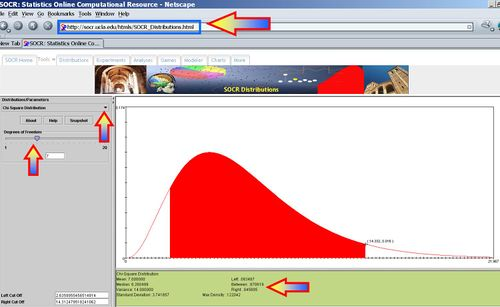Difference between revisions of "AP Statistics Curriculum 2007 Estim Var"

General Advance-Placement (AP) Statistics Curriculum - Estimating Population Variance

In manufacturing, and many other fields, controlling the amount of variance in producing machinery parts is very important. It is important that the parts vary little or not at all.

Estimating Population Variance and Standard Deviation

The most unbiased point estimate for the population variance $$\sigma^2$$ is the sample-variance (s2) and the point estimate for the population standard deviation $$\sigma$$ is the sample standard deviation (s).

We use a Chi-square distribution to construct confidence intervals for the variance and standard distribution. If the process or phenomenon we study generates a Normal random variable, then computing the following random variable (for a sample of size $$n>1$$) has a Chi-square distribution $\chi_o^2 = {(n-1)s^2 \over \sigma^2}$

Chi-square Distribution Properties

• All chi-squares values $$\chi_o^2 \geq 0$$.
• The chi-square distribution is a family of curves, each determined by the degrees of freedom (n-1). See the interactive Chi-Square distribution.
• To form a confidence interval for the variance ($$\sigma^2$$), use the $$\chi^2(df=n-1)$$ distribution with degrees of freedom equal to one less than the sample size.
• The area under each curve of the Chi-square distribution equals one.
• All Chi-square distributions are positively skewed.Approach

Models & strategies for solving the problem, data understanding & inference.

• TBD

Model Validation

Checking/affirming underlying assumptions.

• TBD

• TBD

Examples

Computer simulations and real observed data.

• TBD

Hands-on activities

Step-by-step practice problems.

• TBD